### Stationary groundwater flow

The governing equations of stationary groundwater flow are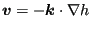(378)

(also called Darcy's law) and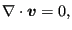(379)

where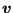is the discharge velocity,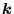is the permeability tensor and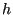is the total head defined by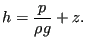(380)

In the latter equation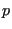is the groundwater pressure,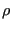is its density and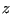is the height with respect to a reference level. The discharge velocity is the quantity of fluid that flows through a unit of total area of the porous medium in a unit of time.

The resulting equation now reads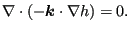(381)

Accordingly, by comparison with the heat equation, the correspondence in Table  (15) arises. Notice that the groundwater flow equation is a steady state equation, and there is no equivalent to the heat capacity term.

 heat groundwater flow T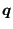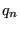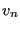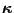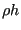0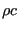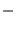Possible boundary conditions are:

1. unpermeable surface under water. Taking the water surface as reference height and denoting the air pressure by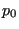one obtains for the total head: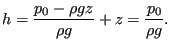(382)

2. surface of seepage, i.e. the interface between ground and air. One obtains: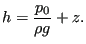(383)

3. unpermeable boundary: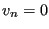4. free surface, i.e. the upper boundary of the groundwater flow within the ground. Here, two conditions must be satisfied: along the free surface one has(384)

In the direction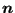perpendicular to the free surfacemust be satisfied. However, the problem is that the exact location of the free surface is not known. It has to be determined iteratively until both equations are satisfied.